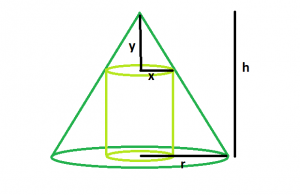GeeksforGeeks App
Open AppBrowser
Continue

# Largest right circular cylinder that can be inscribed within a cone

Given a right circular cylinder which is inscribed in a cone of height h and base radius r. The task is to find the largest possible volume of the cylinder.
Examples:

```Input: r = 4, h = 8
Output: 119.087

Input: r = 5, h = 9
Output: 209.333```Approach: The volume of a cylinder is V = πr^2h
In this problem, first derive an equation for volume using similar triangles in terms of the height and radius of the cone. Once we have the modified the volume equation, we’ll take the derivative of the volume and solve for the largest value.
Let x be the radius of the cylinder and y be the distance from the top of the cone to the top of the inscribed cylinder. Therefore, the height of the cylinder is h – y
The volume of the inscribed cylinder is V = πx^2(h-y)
We use the method of similar ratios to find a relationship between the height and radius, h-y and x
y/x = h/r
y = hx/r
Substitute the equation for y into the equation for volume, V.

V = πx^2(h-y)
V = πx^2(h-hx/r)
V = πx^2h – πx^3h/r
now, dV/dx = d(πx^2h – πx^3h/r)/dx
and setting dV/dx = 0
we get, x = 0, 2r/3
So, x = 2r/3
and, y = 2h/3
So, V = π8r^2h/27

Below is the implementation of the above approach:

## C++

 `// C++ Program to find the biggest``// right circular cylinder that can``// be fit within a right circular cone` `#include ``using` `namespace` `std;` `// Function to find the biggest right circular cylinder``float` `cyl(``float` `r, ``float` `h)``{` `    ``// radius and height cannot be negative``    ``if` `(r < 0 && h < 0)``        ``return` `-1;` `    ``// radius of right circular cylinder``    ``float` `R = (2 * r) / 3;` `    ``// height of right circular cylinder``    ``float` `H = (2 * h) / 3;` `    ``// volume of right circular cylinder``    ``float` `V = 3.14 * ``pow``(R, 2) * H;` `    ``return` `V;``}` `// Driver code``int` `main()``{``    ``float` `r = 4, h = 8;``    ``cout << cyl(r, h) << endl;` `    ``return` `0;``}`

## Java

 `// Java Program to find the biggest``// right circular cylinder that can``// be fit within a right circular cone` `import` `java.io.*;` `class` `GFG {``// Function to find the biggest right circular cylinder``static` `double` `cyl(``double` `r, ``double` `h)``{` `    ``// radius and height cannot be negative``    ``if` `(r < ``0` `&& h < ``0``)``        ``return` `-``1``;` `    ``// radius of right circular cylinder``    ``double` `R = (``2` `* r) / ``3``;` `    ``// height of right circular cylinder``    ``double` `H = (``2` `* h) / ``3``;` `    ``// volume of right circular cylinder``    ``double` `V = ``3.14` `* Math.pow(R, ``2``) * H;` `    ``return` `V;``}` `// Driver code``    ` `    ``public` `static` `void` `main (String[] args) {``    ` `    ``double` `r = ``4``, h = ``8``;``    ``System.out.println (cyl(r, h));``    ``}``//This code is contributed by ajit``}`

## Python 3

 `# Python 3 Program to find the biggest``# right circular cylinder that can``# be fit within a right circular cone``import` `math` `# Function to find the biggest``# right circular cylinder``def` `cyl(r, h):` `    ``# radius and height cannot``    ``# be negative``    ``if` `(r < ``0` `and` `h < ``0``):``        ``return` `-``1` `    ``# radius of right circular cylinder``    ``R ``=` `(``2` `*` `r) ``/` `3` `    ``# height of right circular cylinder``    ``H ``=` `(``2` `*` `h) ``/` `3``    ` `    ``# volume of right circular cylinder``    ``V ``=` `3.14` `*` `math.``pow``(R, ``2``) ``*` `H` `    ``return` `V` `# Driver code``r ``=` `4``; h ``=` `8``;``print``(cyl(r, h), ``"\n"``)` `# This code is contributed``# by Akanksha Rai`

## C#

 `// C# Program to find the biggest``// right circular cylinder that``// can be fit within a right circular cone``using` `System;` `class` `GFG``{``    ` `// Function to find the biggest``// right circular cylinder``static` `double` `cyl(``double` `r, ``double` `h)``{` `    ``// radius and height cannot``    ``// be negative``    ``if` `(r < 0 && h < 0)``        ``return` `-1;` `    ``// radius of right circular cylinder``    ``double` `R = (2 * r) / 3;` `    ``// height of right circular cylinder``    ``double` `H = (2 * h) / 3;` `    ``// volume of right circular cylinder``    ``double` `V = 3.14 * Math.Pow(R, 2) * H;` `    ``return` `V;``}` `// Driver code``static` `public` `void` `Main ()``{``    ``double` `r = 4, h = 8;``    ``Console.WriteLine(cyl(r, h));``}``}` `// This code is contributed by jit_t`

## PHP

 ``

## Javascript

 ``

Output:

`119.087`

Time Complexity: O(1)

Auxiliary Space: O(1)

My Personal Notes arrow_drop_up